# Week 8 - Chemistry Flashcards

Set Details Share
created 3 years ago by SkyRose
5,840 views
Subjects:
chemistry
Page to share:
Embed this setcancel
COPY
code changes based on your size selection
Size:
X

1

The predominant intermolecular force between molecules of I₂ is _____

A) ionic bonds.

B) dipole-dipole interactions.

C) ion-dipole interactions.

D) dispersion forces.

E) covalent bonds.

D) dispersion forces.

2

Which of the following substances would not exhibit any hydrogen bonding interactions in a pure substance?

A) H₂O

B) HF

C) N(CH₃)₃

D) CH₃CH₂OH

E) CH₃NH₂

C) N(CH₃)₃

3

In a molecule of HI, what intermolecular forces are present?

A) dispersion only

B) dipole-dipole only

C) hydrogen bonding only

D) dispersion and dipole-dipole

E) dispersion and hydrogen bonding

D) dispersion and dipole-dipole

4

Which of the following exhibits dipole-dipole intermolecular forces in a pure substance?

A) BF₃

B) CO₂

C) NH₃

D) Br₂

E) CF₄

C) NH₃

5

What intermolecular forces are present between two molecules of CF₃CF₃?

A) dispersion only

B) dipole-dipole only

C) dispersion and dipole-dipole

D) dispersion and hydrogen bonding

E) dipole-dipole and hydrogen bonding

A) dispersion only

6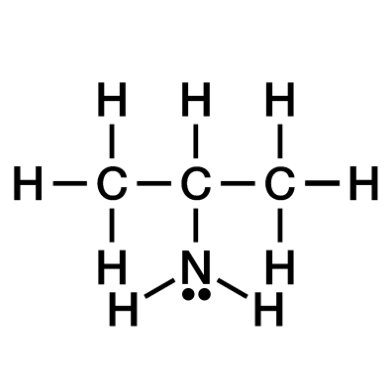Identify the type or types of intermolecular forces present between molecules of the compound pictured below:

A) dispersion only

B) dipole-dipole

C) hydrogen bonding

D) dispersion and dipole-dipole only

E) dispersion, dipole-dipole, and hydrogen bonding

E) dispersion, dipole-dipole, and hydrogen bonding

7

What intermolecular forces are present between two molecules of CH₃CH₂SH?

A) dispersion only

B) dipole-dipole only

C) hydrogen bonding only

D) dispersion and dipole-dipole

E) dispersion and hydrogen bonding

D) dispersion and dipole-dipole

8

Ammonia and hydrogen fluoride both have unusually high boiling points due to _____

A) dispersion forces.

B) dipole-dipole interactions.

C) hydrogen bonding.

D) ion-dipole interactions.

E) low molar masses.

C) hydrogen bonding.

9

Which of the following would you expect to have the highest boiling point?

A) H₂

B) NH₃

C) N₂

D) CH₄

B) NH₃

10

Convert 2.70 atm to torr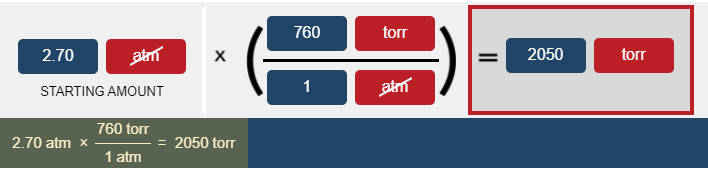The correct answer is 2050 torr.

11

Convert 532 torr to kPa. Remember that multiple steps may be required in the conversion.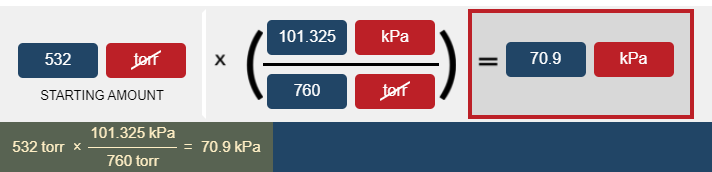The correct answer is 70.9 kPa.

12

The pressure in Denver, Colorado averages about 632 mm Hg. How many atmospheres is this?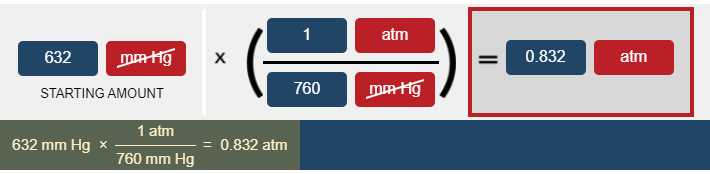The correct answer is 0.832 atm

13

Convert 797 mm Hg to atm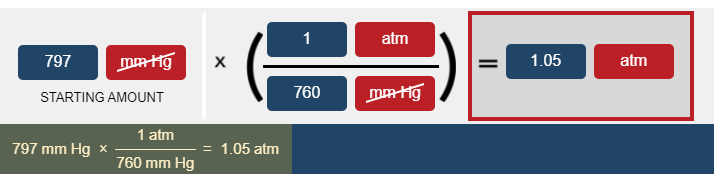The correct answer is 1.05 atm.

14

What is Standard Temperature and Pressure (STP)?

A) 298.15 K and 1.00 atm

B) 273.15 K and 1.00 atm

C) 273.15 K and 0.00 atm

B) 273.15 K and 1.00 atm

15

Convert 750.0 torr to atm.

0.9868 atm (or 1)

16

Which one of the following is the largest value of pressure?

A) 800 torr

B) 950 mmHg

C) 1.10 atm

D) 85.0 kPa

B) 950 mmHg

17

A fixed container containing an ideal gas is heated. The pressure of the gas increases because

A) the gas molecules move faster and strike the walls of the container more often and with more force.

B) the gas molecules slow down, striking the walls of the container less often and with less force.

C) the gas molecules begin sticking together other and strike the walls of the container with twice the force.

D) the gas molecules will melt and fall to the bottom of the container.

E) the gas molecules will no longer behave ideally.

A) the gas molecules move faster and strike the walls of the container more often and with more force.

18

According to the Kinetic Molecular Theory

A) The volume of the gas particles will impact the volume available to the gas.

B) Gas particles exert pressure by colliding with one another

C) The gas particles are moving in circular motions throughout the container.

D) The average kinetic energy is proportional to the temperature of the system.

D) The average kinetic energy is proportional to the temperature of the system.

19

Fundamentally, pressure is defined as force per unit area. What is the source of the force in a gas sample?

A) The force is from gravity acting on the particles.

B) The force is composed of the sum of the collisions only between gas molecules.

C) The force is composed of the sum of the collisions only between the gas molecules and the container.

D) The force is composed of the sum of all collisions--between the gas molecules, each other and the container.

E) The force was imparted to the container in its initial filling, a transfer of momentum.

C) The force is composed of the sum of the collisions only between the gas molecules and the container.

20

Which of the following temperatures is the coldest?

A) 100 K

B) 100 °C

C) 100 °F

A) 100 K

21

A temperature of 103°F or higher is considered a medical emergency. Patient A comes in with a temperature of 38°C. Patient B comes in with a temperature of 313 K. Is either patient having a medical emergency?

A) Yes, patient A only.

B) Yes, patient B only.

C) Yes, both patients.

D) No, neither patient.

E) Not enough information to determine

B) Yes, patient B only.

22

How do you convert a Kelvin temperature to Celsius?

A) Multiply by 9/5 and then add 32

B) Subtract 273.15

C) Subtract by 32, then multiply by 5/9

D) Add 273.15E) Celsius is always the same as Kelvin

B) Subtract 273.15

23

How do you convert a Celsius temperature to Fahrenheit?

A) Multiply by 9/5 and then add 32

B) Subtract 273.15

C) Subtract by 32, then multiply by 5/9

E) Celsius is always the same as Fahrenheit

A) Multiply by 9/5 and then add 32

24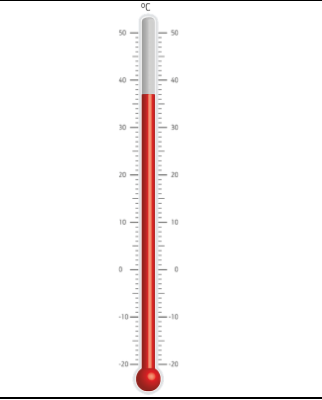Read the thermometer given. What would the temperature be in Kelvin?

A) 300 K

B) 310.2 K

C) -236.2 K

D) 316.2 K

E) 98.6 K

B) 310.2 K

25

A thermometer reads 18.3 °C. What is the temperature in Fahrenheit?

64.9 °F

26

A thermometer reads 111.1 K. What is the temperature in °C?-162.05

-162.1 °C

27

A thermometer reads 155.5 °F. What is the temperature in °C?

68.6 °C

28

While traveling in Europe, you see a weather forecast of 20.0°C. What is this temperature in °F?

68.0 °F

29

A helium balloon initially at room temperature is dunked into a bucket of liquid nitrogen (T = 77 K). Which of the following will occur?

A) Volume increases

B) Volume decreases

C) The number of moles increases

D) The number of moles decreases

E) Temperature increases

B) Volume decreases

30

If the pressure on a movable piston of a closed container is doubled, what will happen?

A) Temperature will double

B) Temperature will double, volume will be cut in half

C) Volume will be cut in half

D) Temperature and volume will double

E) Temperature will increase and volume will decrease, but the specific amount of each cannot be determined with the given information.

E) Temperature will increase and volume will decrease, but the specific amount of each cannot be determined with the given information.

31

If two separate containers A and B have the same volume and temperature, but container A has more gaseous molecules than B, then container A will have:

A) Higher pressure

B) Lower pressure

C) A greater universal gas constant

D) A smaller universal gas constant

A) Higher pressure

32

What gas law states that volume and pressure are inversely proportional at constant temperature and moles?

A) Boyle's Law

B) Charles' Law

C) Gay-Lussac's Law

E) Combined Gas Law

A) Boyle's Law

33

A balloon at 30.0°C has a volume of 222 mL. If the temperature is increased to 53.1°C and the pressure remains constant, what will the new volume be, in mL?

239 mL

34

Which one of the following best describes Charles's Law?

A) The volume of a gas is directly proportional to the temperature of the gas.

B) The pressure of a gas is directly proportional to the temperature of the gas.

C) The volume of a gas is directly proportional to the moles of a gas.

D) The pressure of a gas is inversely proportional to the volume of a gas.

A) The volume of a gas is directly proportional to the temperature of the gas.

35

A 1.20 L weather balloon on the ground has a temperature of 25.0°C and is at atmospheric pressure (1.00 atm). When it rises to an elevation where the pressure is 0.720 atm, then the new volume is 1.80 L. What is the temperature (in °C) of the air at this elevation?

49 °C

36

A weather balloon calibrated at 0.00 °C to have a volume of 20.0 L has what volume in L at -40.0 °C assuming pressure is held constant?

17.1 L

37

One way to measure temperature in some applications is to monitor the gas pressure in a rigid, closed container. What is the temperature (in °C) of such a vessel, calibrated to read 1.00 atm at STP, if the pressure reads 1.25 atm?

68.29 °C

38

The gas in a 250.0 mL piston experiences a change in pressure from 1.00 atm to 2.80 atm. What is the new volume (in mL) assuming the moles of gas and temperature are held constant?

89.3 mL

39

15.0 L of an ideal gas at 298 K and 3.36 atm are heated to 383 K with a new pressure of 6.00 atm. What is the new volume in liters?

10.8 L

40

How many moles of chlorine gas at 120.0 °C and 33.3 atm would occupy a vessel of 12.0 L?

12.4 mol

41

What is the pressure in atm of a 5.00 L tank with 2.50 moles of oxygen at 39.3 °C?

12.8 atm

42

What is the temperature (in K) of 0.300 mole of neon in a 2.00 L vessel at 4.68 atm?

3.80 × 10² K

43

In a 4.00 L pressure cooker, water is brought to a boil. If the final temperature is 115.0 °C at 3.20 atm, how many moles of steam are in the cooker?

0.402 mol

44

What volume in liters would 20.0 moles of sulfur dioxide occupy at 75.3 °C with a pressure of 3.00 atm?

191 L

45

A sample of 2.00 mol of gas in a 10.00 L container is at 45.0 °C. What is the pressure (in atm) of the gas?

5.22 atm